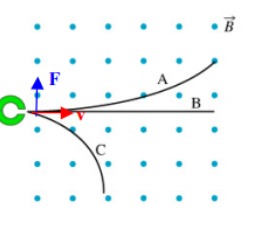# Problem: It is known that a radioactive sample undergoes three different types of radioactive decay and emits three different types of particles: ? decay (helium nuclei), ?? decay (electrons), and ? decay (photons). As shown in the figure, these particles are emitted into a region of space with a uniform magnetic field directed out the page. The particles follow the paths indicated, and none of them bends either into or out of the page. Which type of radioactive decay would produce a decay particle that would move along path A?

###### FREE Expert Solution

The vector v × B points downwards in the magnetic field once the charge enters the region of space.

F is perpendicular to the plane containing both v and B.

81% (302 ratings)###### Problem Details

It is known that a radioactive sample undergoes three different types of radioactive decay and emits three different types of particles: ? decay (helium nuclei), ?? decay (electrons), and ? decay (photons). As shown in the figure, these particles are emitted into a region of space with a uniform magnetic field directed out the page. The particles follow the paths indicated, and none of them bends either into or out of the page. Which type of radioactive decay would produce a decay particle that would move along path A?